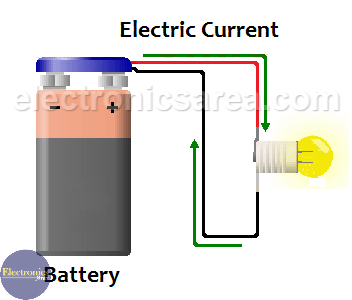# What is Voltage?

Voltage is the electrical potential difference caused by the accumulation of electric charges on a point, or a material. If we remove electrons from a material, its total electric charge will be positive. Remember that we are removing electrons, which are negatively charged, from a neutral atom which has no charge.

This causes the atom is no longer neutral but have a positive charge. We can see that there are 6 protons in the atom (positive charge) and 4 electrons (negative charge). In conclusion, the total charge is positive.

If we increase the amount of electrons in a material, its total electric charge will be negative. We can see that there are 6 protons in the atom (positive charge) and 8 electrons (negative charge). In conclusion, the total electric charge is negative.

If we have two materials with different levels of electric charges, then we say that there is a potential difference between them. To change the electric charge of a material it is necessary to apply energy to the atom. There are several methods for doing this:

• friction
• pressure
• heat
• by magnetism
• by chemical action

When two points having a potential difference are linked through a conductor, it produces an electric current flow. The electric current flows from the point with the greatest potential, to the point with lower potential. This is the case of a voltage source.From the above we can find another more simple explanation: “Voltage is a kind of force that push charges through a conductor”. The larger the potential difference, the larger the force.

So, to get a lamp like the one in the figure to light up, a current must flow through the wires to which it is connected. For this to happen there must be a force called electromotive force (EMF) or to better understand a “voltage”.

Unit of voltage is volts.

## Some voltage unit conversions

• 1 kilovolt = 1000 volts.
• 1 kilovolt = 1000000 microvolts.
• 1 microvolt = 1 x 10-6 volts.
• 1 millivolt = 1/1000 = 0.001 volts = 1 x 10-3 volts.
• 1 Megavolt = 1000 000 volts = 1 x 106 volts.
• 1 Megavolt = 1000 kilovolts.
•
•
•
•
•
•
•
•
•
•
•
•
•
•
•
•
•
•